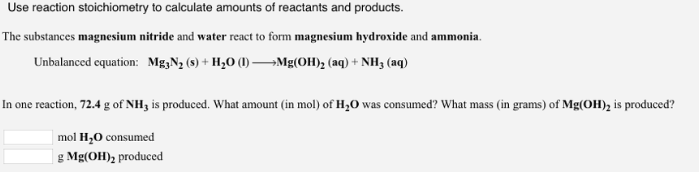# Problem: Use reaction stoichiometry to calculate amounts of reactants and products. The substances magnesium nitride and water react to form magnesium hydroxide and ammonia. Unbalanced equation: Mg3N2 (s) + H2O (I) → Mg(OH)2 (aq) + NH3 (aq) In one reaction, 72.4 g of NH3 is produced. What amount (in mol) of H2O was consumed? What mass (in grams) of Mg(OH)2 is produced?

###### FREE Expert Solution
83% (41 ratings)###### Problem Details

Use reaction stoichiometry to calculate amounts of reactants and products.

The substances magnesium nitride and water react to form magnesium hydroxide and ammonia.

Unbalanced equation: Mg3N2 (s) + H2O (I) → Mg(OH)2 (aq) + NH3 (aq)

In one reaction, 72.4 g of NH3 is produced. What amount (in mol) of H2O was consumed? What mass (in grams) of Mg(OH)2 is produced?